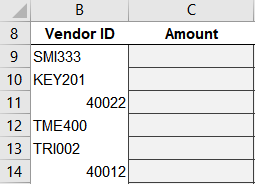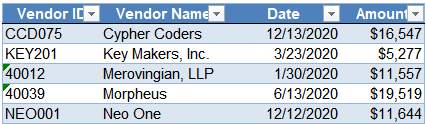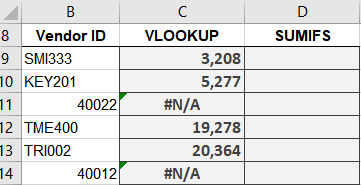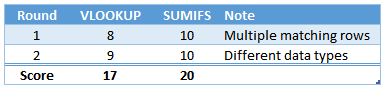# Battle of Heavyweights: Round 2Round 2 begins! In this round, the goal is to understand what happens when values that are supposed to match are stored as different data types. Different data types? Cells can store many kinds of data, including numbers, text, dates, times, and so on. The format you apply to a cell doesn’t impact the data type of the stored cell value. Two cells may therefore appear to have the same value, but, one cell may store it as a number and the other cell may store it as a text string. So, the question is: do VLOOKUP and SUMIFS match equivalent values when they are stored as different data types? Let’s find out. Round 2 begins now!

## Round 2

Let’s see a concrete example of data types.

We have a column of Vendor IDs, and we want to retrieve the amount for each:Some of the Vendor IDs, like SMI333 and KEY201, are text strings. They look like text strings and are stored as text strings. In fact, the default cell alignment for text values is left-aligned. We can of course change that manually, but by default, Excel left-aligns text values.

We also have some Vendor IDs that are numeric, such as 40022 and 40012. These look like numbers and are stored as numbers. The default cell alignment for numbers is right-aligned, and these are in fact right-aligned. So far so good.

Now let’s take a look at our data table:Interesting … here the Vendor IDs are all left-aligned, including 40012 and 40039. So … what’s up? Are they text strings or numbers? This brings us to the exact point of this post. To us, they look like numbers … but … Excel has stored them as text values. This can happen depending on the data source and how we get the data into Excel.

In fact, as we examine the data table, we see that the numeric ids have a green alert on the cell. The alerts are telling us that these values appear to be numbers but are actually stored as text strings. They look like numbers, but the data type is text … and even if we apply some type of cell format, or right-align them, they will still be stored as text.

So, the question is, will they match? Specifically, will equivalent values match between the lists, even when stored as different data types?

Let’s find out.

## VLOOKUP

We try first with VLOOKUP. We write the following formula into C9:

`=VLOOKUP(B9, Table1, 4, 0)`

We fill it down, and … hmmm:The text IDs work well, but we get an error for the numeric IDs. And, that is exactly right: VLOOKUP does not match equivalent values when stored as different data types.

What about SUMIFS? Let’s find out.

## SUMIFS

We write the following formula into D9:

`=SUMIFS(Table1[Amount], Table1[Vendor ID], B9)`

We fill the formula down, and bam:Wow … it worked! So, here is the deal: SUMIFS will match equivalent values, even when stored as different data types.

Way to go SUMIFS!

## Analysis

So, here is the key point of this post:

VLOOKUP does not match equivalent values when stored as different data types;

SUMIFS does

In practice, which function would you use when trying to complete a task like the one presented? Me to … SUMIFS!

Let’s award the points and update our scoreboard. The “winner” of the round gets 10 points, so, 10 points to SUMIFS. This reminds us that when there are different data types in the lookup column, SUMIFS works just fine.

And let’s think about VLOOKUP. Could VLOOKUP accomplish this task with a helper function, or is it knocked down? Well, we could use a nested helper function like VALUE/TEXT to change the data type as discussed here. We could also manually change the data type with a variety of approaches. So, we’ll award 9 points to VLOOKUP.

So, in this round, we see a score of 10 for SUMIFS, and 9 for VLOOKUP. These scores remind us that when equivalent values are stored as different data types, SUMIFS will work just fine and that VLOOKUP can work with a bit of assistance.

Here is a recap per round so far:And let’s update the scoreboard please …Alright … that is it for this round, see you in round 3!

Sample file: Round2.xlsx

Get a quick email notice when a new Excel article is available

• This field is for validation purposes and should be left unchanged.